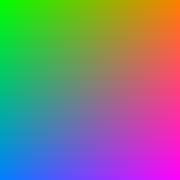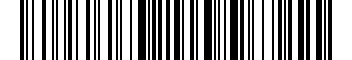< Back# Dynamically Generating BMP Files

Written: 2012-04-19

## Introduction

Occasionally there arises the need to be able to dynamically generate images or graphics using a script instead of creating the images using an image editing program such as Adobe Photoshop.

One possible application for generating dynamic images is creating bar codes. Data, such as a number or text string, is encoded and displayed as an image that can then be read by a bar code reader. Creating such a bar code image would be very tedious using Photoshop, but is quite simple using an appropriate script.In this article, I'll discuss the basics of creating a BMP (bitmap image file) format graphic. I'll be giving example code in Perl, but it should be trivial to apply the principles to any other programming language.

## Addressing the Use of Graphics Libraries

There are graphics libraries available for this kind of thing, so you could avoid ever having to know anything about the BMP file format if you want. However, there are reasons why using a graphics library is not an option, such as:

• You need a faster, light-weight solution.
• You don't have an appropriate library available and are not able to install one.
• Your solution needs to minimize the number of dependencies.

## The BMP File Format Details

The BMP file format is discussed in depth on many websites, so I will not repeat all that here. Instead I will demonstrate a working example of creating a 24-bit color BMP image using Perl.

## File Size and Row Padding

Since we are creating a 24-bit color image, there will be 3-bytes per pixel. This would indicate that one row of pixels would require `(3 * \$width)` bytes of space. However, the BMP file format requires that each row of pixels take up an exact multiple of 4-bytes. Therefore, one row of pixels might need some padding at the end:

```my \$row_padding = (3 * \$width) % 4;
```

Now, the number of bytes per row is equal to the bytes needed for one row of pixels, plus the padding:

```my \$row_size = 3 * \$width + \$row_padding
```

And the total amount of bytes needed for all rows is:

```my \$data_size = \$height * \$row_size;
```

The length of the headers in a BMP file can vary, but the headers in our basic 24-bit color BMP image require 54 bytes. We can now calculate the total file size:

```my \$header_size = 54;
my \$file_size = \$header_size + \$data_size;
```

## File Handle and Binmode

In this example we will be writing the image data out to the STDOUT output stream. Since it may also be useful to be able to write the data directly to a file, we will use a filehandle variable, `\$fh`, to allow the stream to be changed easily.

```my \$fh = \*STDOUT;
binmode(\$fh);
```

## Bitmap File Header

The Bitmap File Header is written first. This header is 14-bytes long:

```print \$fh "\x42\x4D";               # BMP magic number
print \$fh pack("V", \$file_size);
print \$fh "\0\0\0\0";               # Reserved
print \$fh pack("V", \$header_size);  # Offset to the image data
```

In Perl, the pack("V", ...) command encodes the value as a little-endian 32-bit (4-byte) unsigned binary number.

Next comes the DIB Header, which contains information such as the image width, image height and number of colors. The DIB Header size can vary, depending on whether the BMP file contains ICC color profile information or gamma information, but we will write a simple 40-byte DIB header:

```print \$fh "\x28\0\0\0";          # Header size (40 bytes)
print \$fh pack("V", \$width);     # Bitmap width
print \$fh pack("V", \$height);    # Bitmap height
print \$fh "\1\0";                # Number of color planes
print \$fh "\x18\0";              # Bits per pixel (24-bits)
print \$fh "\0\0\0\0";            # Compression method
print \$fh pack("V", \$data_size); # Image data size
print \$fh "\x13\x0B\0\0";        # Horizontal resolution (px/m)
print \$fh "\x13\x0B\0\0";        # Vertical resolution (px/m)
print \$fh "\0\0\0\0";            # Number of colors in palette
print \$fh "\0\0\0\0";            # Number of important colors
```

We will not be compressing the data, so the compression method is set to 0. The horizontal and vertical resolutions are set to 2835 pixels per meter, which is 72 pixels per inch.

## Image Data

Finally, each row of image data is written with the appropriate padding added at the end of each row. Note that the image is written with the bottom row first and the top row last:

```# Start of bitmap data;
for (my \$y = \$height - 1; \$y >= 0; \$y = \$y - 1)
{
for (my \$x = 0; \$x < \$width; \$x = \$x + 1)
{
# Function returns 24-bit pixel value
# Color order must be (Blue, Green, Red)
print \$fh generate_image_data(\$x, \$y);
}

for (my \$p = 0; \$p < \$row_padding; \$p = \$p + 1)
{
print \$fh "\0";
}
}
```

We use a generic `generate_image_data()` function which returns the 24-bit RGB value for a particular (x, y) coordinate in the graphic. This function can be customized to generate the desired image.

## Putting It Together

Combining all the code and adding an appropriate `generate_image_data()` function, we can write a Perl script that generates a colorful gradient BMP image. If the script is being run as a CGI script, then a BMP HTTP header is written out first:

```#!/usr/bin/perl

use strict;
use CGI;

my \$width = 180;
my \$height = 180;

my \$row_padding = (3 * \$width) % 4;
my \$row_size = 3 * \$width + \$row_padding
my \$data_size = \$height * \$row_size;

my \$header_size = 54;
my \$file_size = \$header_size + \$data_size;

if (\$ENV{'REQUEST_METHOD'})
{
-type => 'image/bmp',
-Content_length => \$file_size,
-'content-disposition' => 'inline; filename="image.bmp"',
-filename => 'image.bmp'
);
}

my \$fh = \*STDOUT;
binmode(\$fh);

print \$fh "\x42\x4D";               # BMP magic number
print \$fh pack("V", \$file_size);
print \$fh "\0\0\0\0";               # Reserved
print \$fh pack("V", \$header_size);  # Offset to the image data

print \$fh "\x28\0\0\0";          # Header size (40 bytes)
print \$fh pack("V", \$width);     # Bitmap width
print \$fh pack("V", \$height);    # Bitmap height
print \$fh "\1\0";                # Number of color planes
print \$fh "\x18\0";              # Bits per pixel (24-bits)
print \$fh "\0\0\0\0";            # Compression method
print \$fh pack("V", \$data_size); # Image data size
print \$fh "\x13\x0B\0\0";        # Horizontal resolution (px/m)
print \$fh "\x13\x0B\0\0";        # Vertical resolution (px/m)
print \$fh "\0\0\0\0";            # Number of colors in palette
print \$fh "\0\0\0\0";            # Number of important colors

# Start of bitmap data
for (my \$y = \$height - 1; \$y >= 0; \$y = \$y - 1)
{
for (my \$x = 0; \$x < \$width; \$x = \$x + 1)
{
# Function returns 24-bit pixel value
# Color order must be (Blue, Green, Red)
print \$fh generate_image_data(\$x, \$y);
}

for (my \$p = 0; \$p < \$row_padding; \$p = \$p + 1)
{
print \$fh "\0";
}
}

sub generate_image_data
{
my(\$x, \$y) = @_;

\$x /= \$width;
\$y /= \$height;

# Generate some pretty colors
my \$R = pack("C", 255 * \$x);
my \$B = pack("C", 255 * \$y);
my \$G = pack("C", int(255 * (1 - ((\$x + \$y)/2))));

return \$B . \$G . \$R;
}
```